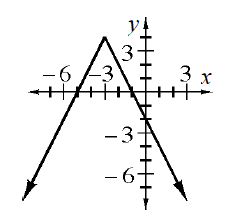### Home > CCA2 > Chapter 2 > Lesson 2.2.5 > Problem2-164

2-164.

Use your knowledge of absolute value functions to find the equation of the graph at right.

What shape is the graph?
Which direction does it open?
Where is the vertex?
How 'steep' is the graph?

$y=-2\left | x+3 \right |+4$